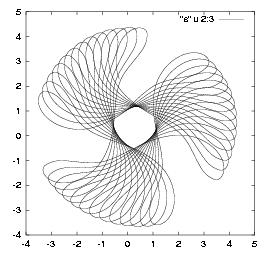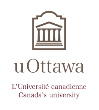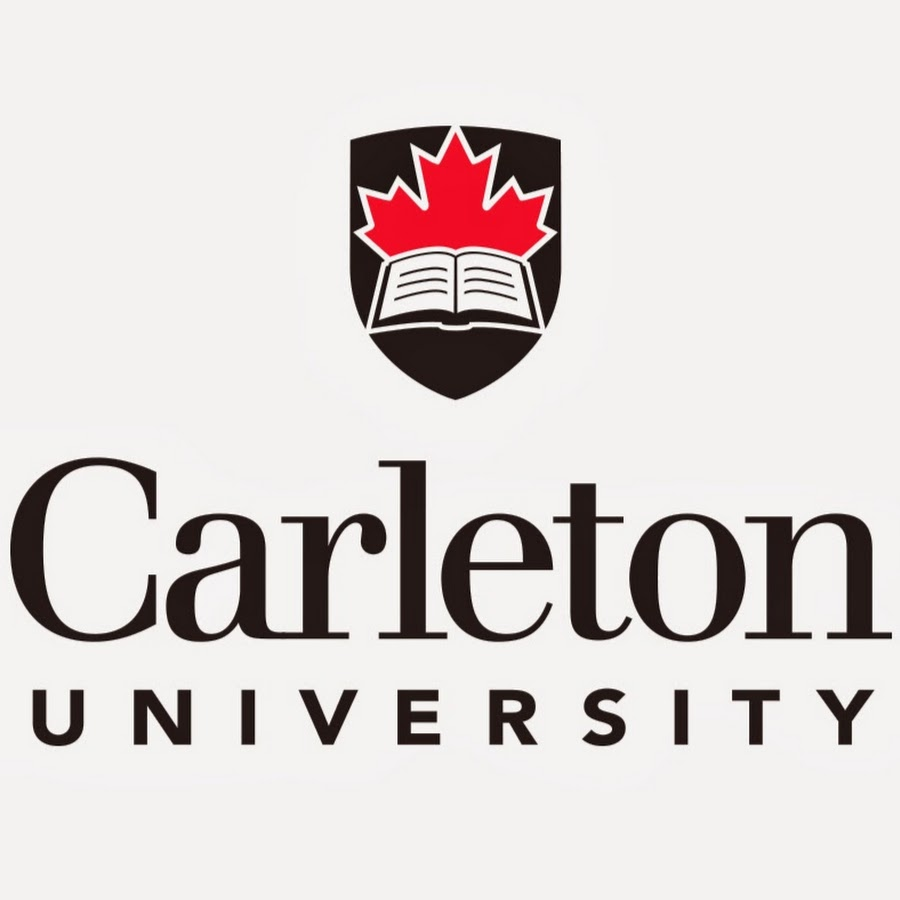# Applied Mathematics Ottawa

## Ottawa, October 2nd, 2020

 Name Research Area David Amundsen Nonlinear PDEs Yves Bourgault Computational fluid dynamics, image processing Lucy Campbell Fluid dynamics Benoit Dionne Bifurcation theory, symmetry Diane Guignard Numerical analysis, partial differential equations uncertainty quantification Victor LeBlanc Bifurcation theory, spiral waves Emmanuel Lorin Numerical analysis, quantum chemistry Frithjof Lutscher Mathematical biology, spatial ecology Sam Melkonian Nonlinear Differential Equations, Fluid Mechanics Angelo Mingarelli Ordinary Differential Equations, Difference Equations Abbas Momeni Analysis, optimal transport, parital differential equations Arian Novruzi Shape optimization, PDEs, numerical analysis, mathematical modeling Tanya Schmah Data science, geometric mechanics, diffeomorphic image registration Robert Smith? Mathematical biology, infectious diseases Cristina Stoica Applied dynamical systems, geometric mechanics

The group meets regularly for its Applied Mathematics Seminar.
If you want to be included in the email list for this seminar,